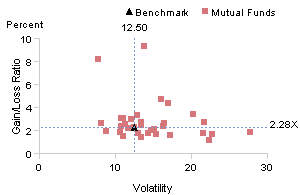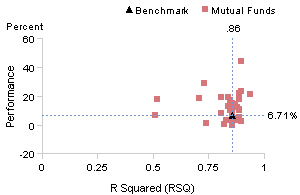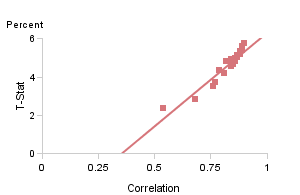# KavaChart Gallery .. Scatter Plots

 Annualized Relative Average Performance Global Equity Mutual Funds Annualized Gain/Loss Ratio Global Equity Mutual FundsActively managed funds may increase volatility (risk) and risk should be compensated for by greater returns relative to a benchmark. Tracking error is the degree to which fund volatility differs from its benchmark. Performance is measured relative to the benchmark. Gain/Loss ratio is simply the sum of positive gains each month divided by the sum of the losses. Volatility is a measure of risk. One might expect high and low gain loss ratios to be associated with relatively high volatility. Annualized Average Performance Global Equituy Mutual Funds Annualized Correlation Global Equity Mutual FundsR-squared (RSQ) is a statistical measure used to estimate the degree to which a fund's performance is explained by the performance of a benchmark. For example a fund with a RSQ of .86 relative to the S&P 500 means that 86% of the funds historical performance can be explained by the performance of the S&P 500. Correlation is a measure of how two variables behave relative to one another. Funds whose performance moves together over time are correlated. T-stat is a measure of the reliability of the correlation (or correlation coefficient). This chart indicates, with a farily high degree of reliability that the performance of these funds is positively correlated and as a group they will move in the same direction.